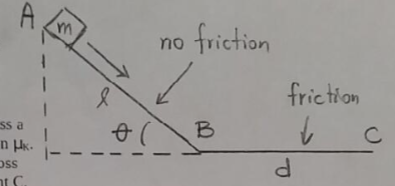# Problem: A box of mass m = 1.80 kg is at point A, which is at the top of an inclined plane of length ℓ = 2.40 m and inclination angle θ = 36.9°. The inclined angle is greased so that it is frictionless. The box slides down the inclined plane to the point B, where it starts to move horizontally across a surface with coefficient of kinetic friction μk. The box moves a distance d = 4.80 m across this surface before coming to rest at point C. Find the gravitational potential energy of the box at point A (the top of the incline).

###### Problem Details

A box of mass m = 1.80 kg is at point A, which is at the top of an inclined plane of length ℓ = 2.40 m and inclination angle θ = 36.9°. The inclined angle is greased so that it is frictionless. The box slides down the inclined plane to the point B, where it starts to move horizontally across a surface with coefficient of kinetic friction μk. The box moves a distance d = 4.80 m across this surface before coming to rest at point C.

Find the gravitational potential energy of the box at point A (the top of the incline).